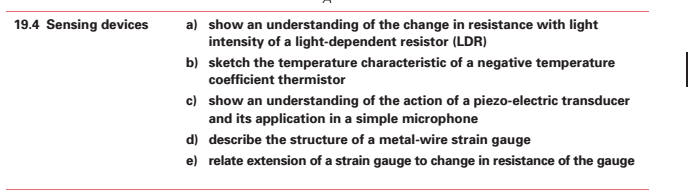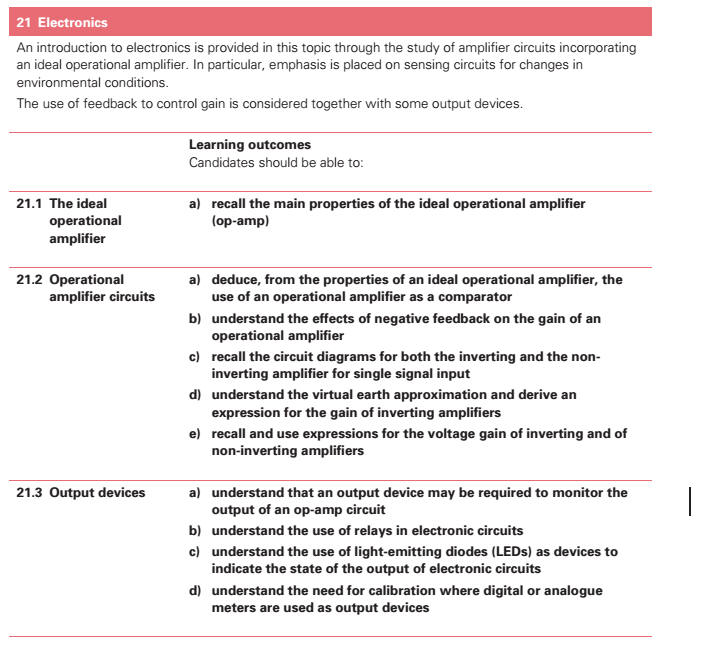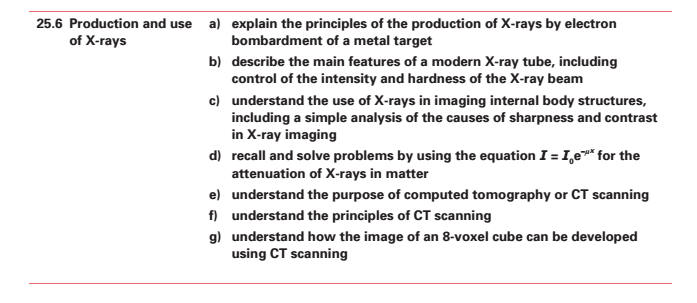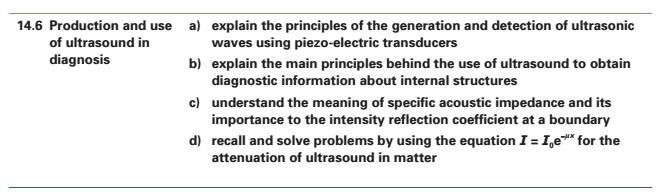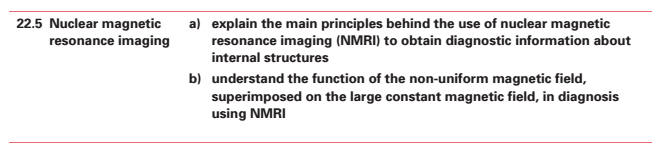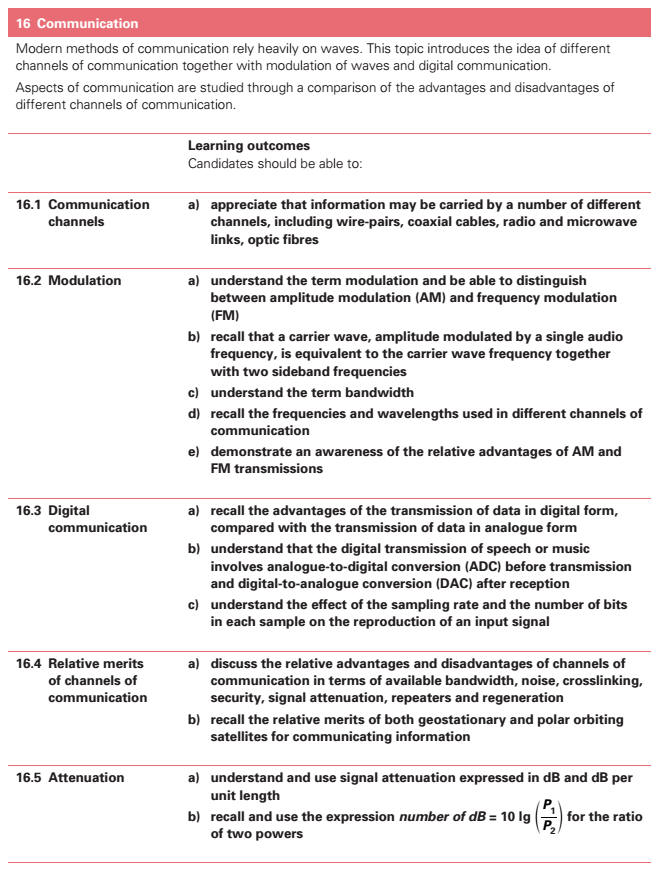APPLICATIONS OF PHYSICS - GATHERING AND COMMUNICATING INFORMATION  most of this is now inherent throughout the syllabus Direct Sensing (a) show an understanding that an electronic sensor consists of a sensing device and a circuit that provides an output voltage. (b) show an understanding of the change in resistance[][] with light intensity of a light dependent resistor (LDR). (c) sketch the temperature[] characteristic of a negative temperature[] coefficient thermistor[]. (d) show an understanding of the action of a piezo-electric transducer and its application in a simple microphone. (e) describe the structure of a metal wire strain gauge. (f) relate extension of a strain gauge[] to change in resistance[][] of the gauge. (g) show an understanding that the output from sensing devices can be registered as a voltage. (h) recall the main properties of the ideal operational amplifier (op-amp). (i) deduce, from the properties of an ideal operational amplifier, the use of an operational amplifier as a comparator. (j) show an understanding of the effects of negative feedback on the gain of an operational amplifier. (k) recall the circuit diagrams for both the inverting and the non-inverting amplifier for single signal input. (l) show an understanding of the virtual earth approximation and derive an expression for the gain of inverting amplifiers. (m) recall and use expressions for the voltage gain of inverting and of non-inverting amplifiers. (n) show an understanding of the use of relays in electronic circuits[]. (o) show an understanding of the use of light-emitting diodes (LEDs) as devices to indicate the state of the output of electronic circuits[]. (p) show an understanding of the need for calibration[] where digital or analogue meters are used as output devices. Remote Sensing (b) explain the principles of the production of X-rays by electron bombardment of a metal target. (c) describe the main features of a modern X-ray tube, including control of the intensity and hardness of the X-ray beam. (d) show an understanding of the use of X-rays in imaging internal body structures, including a simple analysis of the causes of sharpness and contrast in X-ray imaging. (e) recall and solve problems by using the equation I = I0e-μx for the attenuation of Xrays in matter. (f) show an understanding of the purpose of computed tomography or CT scanning. (g) show an understanding of the principles of CT scanning. (h) show an understanding of how the image of an 8-voxel cube can be developed using CT scanning. (i) explain the principles of the generation and detection of ultrasonic waves using piezo-electric transducers. (j) explain the main principles behind the use of ultrasound to obtain diagnostic information about internal structures. (k) show an understanding of the meaning of acoustic impedance and its importance to the intensity[] reflection coefficient at a boundary. (l) explain the main principles behind the use of magnetic resonance[] to obtain diagnostic information about internal structures. (m) show an understanding of the function of the non-uniform magnetic field, superimposed on the large constant magnetic field, in diagnosis using magnetic resonance. Communicating Information (a) understand the term modulation and be able to distinguish between amplitude modulation (AM) and frequency modulation (FM). (b) recall that a carrier wave, amplitude modulated by a single audio frequency, is equivalent to the carrier wave frequency together with two sideband frequencies. (c) understand the term bandwidth. (d) demonstrate an awareness of the relative advantages of AM and FM transmissions. (e) recall the advantages of the transmission of data in digital form. (f) understand that the digital transmission of speech or music involves analogue-to-digital conversion (ACD) on transmission and digital-to-analogue conversion (DAC) on reception. (g) show an understanding of the effect of the sampling rate and the number of bits in each sample on the reproduction of an input signal. (h) appreciate that information may be carried by a number of different channels, including wire-pairs, coaxial cables, radio and microwave links, and optic fibres. (i) discuss the relative advantages and disadvantages of channels of communication in terms of available bandwidth, noise, cross-linking, security, signal attenuation, repeaters and regeneration, cost and convenience. (j) describe the use of satellites in communication. (k) recall the relative merits of both geostationary and polar orbiting satellites for communicating information. (l) recall the frequencies and wavelengths used in different channels of communication. (m) understand and use signal attenuation expressed in dB and dB per unit length. (n) recall and use the expression number of dB = 10 lg(P1/P2) for the ratio of two powers.